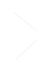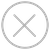TensorSpace.jsTensorSpace ConverterTensorSpace Converter API
TensorSpace-Converter 示例脚本：
``````\$ tensorspacejs_converter \
--input_model_from="XXX" \
--input_model_format="YYY" \
--output_layer_names="EEE1,EEE2,EEE3" \
input_path \
output_path``````

Positional Arguments 介绍
input_path 模型输入路径，TensorSpace-Converter 将会读取该路径中的模型进行转化
output_path 模型输出路径（文件夹），TensorSpace-Converter 会将经过转化的模型输出到该路径中

input_model_from 配置模型是使用哪种深度学习库训练并保存的，如果模型是使用TensorFlow 训练并保存的的，配置 tensorflow，如果模型是使用 Keras 训练并保存的，配置 keras，如果模型是使用 TensorFlow.js 训练并保存的，配置 tfjs
input_model_format 模型的格式。
output_layer_names 输出希望在TensorSpace 中可视化的 layer，使用英文半角逗号“,”分割。